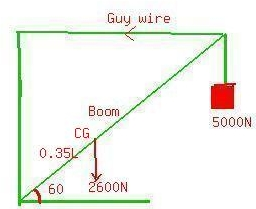# The boom weighs 2600N. The boom is attached with a frictionless pivot at its lower end. It is not uniform; the distance of its center of gravity fromwaigaK 2020-12-01 Answered
The boom weighs 2600N. The boom is attached with a frictionless pivot at its lower end. It is not uniform; the distance of its center of gravity from the pivot is 35% of its length. a) Find the tension in the guy wire and the horizontal and vertical components of the force exerted on the boom at its lower end. b) Does the line of action of the force exerted on the boom a tits lower end lie along the boom?
You can still ask an expert for help

• Questions are typically answered in as fast as 30 minutes

Solve your problem for the price of one coffee

• Math expert for every subject
• Pay only if we can solve itElberteTaking torques about the pivot point A
$TL\mathrm{sin}60=5000L\mathrm{cos}60+2600\left(0.35L\right)\mathrm{cos}60$
or

The vertical force exerted on the boon by the pivot

the Horizontal force

No, $\mathrm{tan}\left(\frac{{F}_{v}}{{F}_{h}}\right)$ is not equal to zero